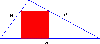BrainDen.com - Brain Teasers
• 0

# Square inscribed in a triangle

Go to solution Solved by ThunderCloud,

## Question

A triangle has sides 10, 17, and 21. A square is inscribed in the triangle. One side of the square lies on the longest side of the triangle. The other two vertices of the square touch the two shorter sides of the triangle. What is the length of the side of the square?## Recommended Posts

• 0
• Solution

The length of the side is 168 / 29. Reasoning: the triangle atop the square is similar to the triangle it is inscribed within, and so it has the same proportions. The two "lower" triangles are both right triangles and so the Pythagorean theorem applies. Call the square's length

x, the length along the long side of the triangle to the left of the square y and the length to the right of the square z so that x + y + z = 21. Using Pythagoras, and the similarity in triangle proportions, we also have that x^2 + y^2 = (10 - (10/21)*x)^2 and similarly that x^2 + z^2 = (17 - (17/21)*x)^2. Much further algebra yields the above result.
##### Share on other sites
• 0

spolier doesnt seem to work..

33.55??

##### Share on other sites
• 0

The area of the big triangle (S) is a sum of area of the small traingle (T) atop of the square and the area of trapezoid (let's call it M):

S = M + T.
Triangle T is similar to S, so:
T/S = (a/21)^2.
(where a denotes side of the square).
Area of trapezoid M is:
M = (21+a)*a/2.
Heron's formula tor triangle S gives:
S = 84.
84 = (21+a)*a/2 + 84*(a/21)^2
that has one positive solution:
a = 168/29.
##### Share on other sites
• 0

The length of the side is 168 / 29. Reasoning: the triangle atop the square is similar to the triangle it is inscribed within, and so it has the same proportions. The two "lower" triangles are both right triangles and so the Pythagorean theorem applies. Call the square's length

x, the length along the long side of the triangle to the left of the square y and the length to the right of the square z so that x + y + z = 21. Using Pythagoras, and the similarity in triangle proportions, we also have that x^2 + y^2 = (10 - (10/21)*x)^2 and similarly that x^2 + z^2 = (17 - (17/21)*x)^2. Much further algebra yields the above result.

It always amazes me when people use strategies hidden in the problem that i didn't even consider and even more makes me jealous when their strategy is more efficient than my own.

##### Share on other sites
• 0

The length of the side is 168 / 29. Reasoning: the triangle atop the square is similar to the triangle it is inscribed within, and so it has the same proportions. The two "lower" triangles are both right triangles and so the Pythagorean theorem applies. Call the square's length

x, the length along the long side of the triangle to the left of the square y and the length to the right of the square z so that x + y + z = 21. Using Pythagoras, and the similarity in triangle proportions, we also have that x^2 + y^2 = (10 - (10/21)*x)^2 and similarly that x^2 + z^2 = (17 - (17/21)*x)^2. Much further algebra yields the above result.

It always amazes me when people use strategies hidden in the problem that i didn't even consider and even more makes me jealous when their strategy is more efficient than my own.

Thanks.Although witzar's answer involves even less work than mine.

And I was actually not aware of Heron's formula, so I learned something.## Join the conversation

You can post now and register later. If you have an account, sign in now to post with your account.×   Pasted as rich text.   Paste as plain text instead

Only 75 emoji are allowed.

×   Your previous content has been restored.   Clear editor

×   You cannot paste images directly. Upload or insert images from URL.